# pandas.plotting.boxplot¶

pandas.plotting.boxplot(data, column=None, by=None, ax=None, fontsize=None, rot=0, grid=True, figsize=None, layout=None, return_type=None, **kwargs)[source]

Make a box plot from DataFrame columns.

Make a box-and-whisker plot from DataFrame columns, optionally grouped by some other columns. A box plot is a method for graphically depicting groups of numerical data through their quartiles. The box extends from the Q1 to Q3 quartile values of the data, with a line at the median (Q2). The whiskers extend from the edges of box to show the range of the data. The position of the whiskers is set by default to 1.5 * IQR (IQR = Q3 - Q1) from the edges of the box. Outlier points are those past the end of the whiskers.

For further details see Wikipedia’s entry for boxplot.

Parameters
columnstr or list of str, optional

Column name or list of names, or vector. Can be any valid input to pandas.DataFrame.groupby().

bystr or array-like, optional

Column in the DataFrame to pandas.DataFrame.groupby(). One box-plot will be done per value of columns in by.

axobject of class matplotlib.axes.Axes, optional

The matplotlib axes to be used by boxplot.

fontsizefloat or str

Tick label font size in points or as a string (e.g., large).

rotint or float, default 0

The rotation angle of labels (in degrees) with respect to the screen coordinate system.

gridbool, default True

Setting this to True will show the grid.

figsizeA tuple (width, height) in inches

The size of the figure to create in matplotlib.

layouttuple (rows, columns), optional

For example, (3, 5) will display the subplots using 3 columns and 5 rows, starting from the top-left.

return_type{‘axes’, ‘dict’, ‘both’} or None, default ‘axes’

The kind of object to return. The default is axes.

• ‘axes’ returns the matplotlib axes the boxplot is drawn on.

• ‘dict’ returns a dictionary whose values are the matplotlib Lines of the boxplot.

• ‘both’ returns a namedtuple with the axes and dict.

• when grouping with by, a Series mapping columns to return_type is returned.

If return_type is None, a NumPy array of axes with the same shape as layout is returned.

**kwargs

All other plotting keyword arguments to be passed to matplotlib.pyplot.boxplot().

Returns
result

See Notes.

Series.plot.hist

Make a histogram.

matplotlib.pyplot.boxplot

Matplotlib equivalent plot.

Notes

The return type depends on the return_type parameter:

• ‘axes’ : object of class matplotlib.axes.Axes

• ‘dict’ : dict of matplotlib.lines.Line2D objects

• ‘both’ : a namedtuple with structure (ax, lines)

For data grouped with by, return a Series of the above or a numpy array:

Use return_type='dict' when you want to tweak the appearance of the lines after plotting. In this case a dict containing the Lines making up the boxes, caps, fliers, medians, and whiskers is returned.

Examples

Boxplots can be created for every column in the dataframe by df.boxplot() or indicating the columns to be used:

>>> np.random.seed(1234)
>>> df = pd.DataFrame(np.random.randn(10, 4),
...                   columns=['Col1', 'Col2', 'Col3', 'Col4'])
>>> boxplot = df.boxplot(column=['Col1', 'Col2', 'Col3'])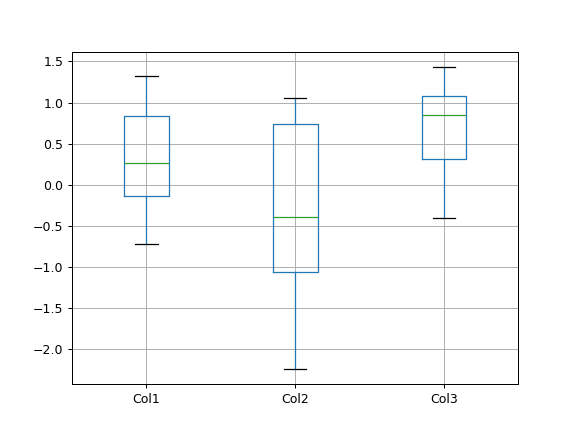Boxplots of variables distributions grouped by the values of a third variable can be created using the option by. For instance:

>>> df = pd.DataFrame(np.random.randn(10, 2),
...                   columns=['Col1', 'Col2'])
>>> df['X'] = pd.Series(['A', 'A', 'A', 'A', 'A',
...                      'B', 'B', 'B', 'B', 'B'])
>>> boxplot = df.boxplot(by='X')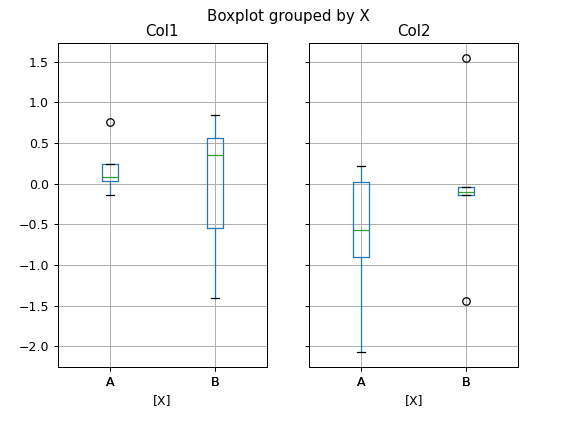A list of strings (i.e. ['X', 'Y']) can be passed to boxplot in order to group the data by combination of the variables in the x-axis:

>>> df = pd.DataFrame(np.random.randn(10, 3),
...                   columns=['Col1', 'Col2', 'Col3'])
>>> df['X'] = pd.Series(['A', 'A', 'A', 'A', 'A',
...                      'B', 'B', 'B', 'B', 'B'])
>>> df['Y'] = pd.Series(['A', 'B', 'A', 'B', 'A',
...                      'B', 'A', 'B', 'A', 'B'])
>>> boxplot = df.boxplot(column=['Col1', 'Col2'], by=['X', 'Y'])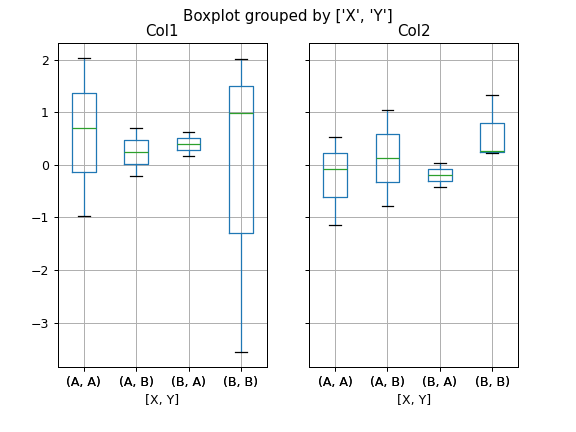The layout of boxplot can be adjusted giving a tuple to layout:

>>> boxplot = df.boxplot(column=['Col1', 'Col2'], by='X',
...                      layout=(2, 1))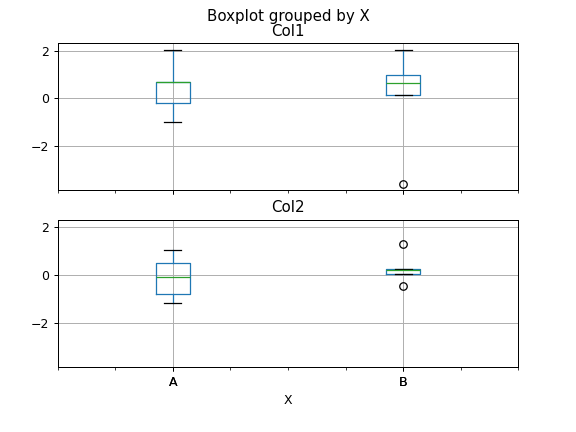Additional formatting can be done to the boxplot, like suppressing the grid (grid=False), rotating the labels in the x-axis (i.e. rot=45) or changing the fontsize (i.e. fontsize=15):

>>> boxplot = df.boxplot(grid=False, rot=45, fontsize=15)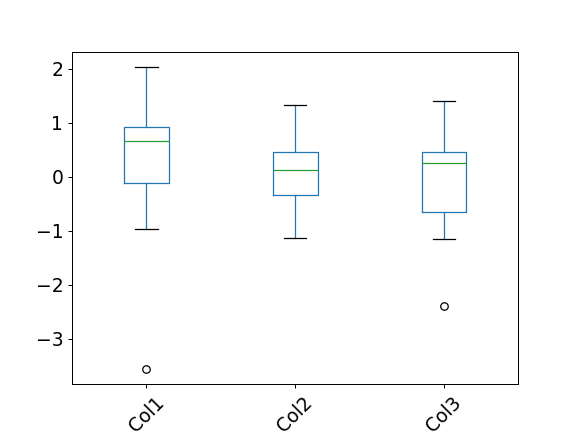The parameter return_type can be used to select the type of element returned by boxplot. When return_type='axes' is selected, the matplotlib axes on which the boxplot is drawn are returned:

>>> boxplot = df.boxplot(column=['Col1', 'Col2'], return_type='axes')
>>> type(boxplot)
<class 'matplotlib.axes._subplots.AxesSubplot'>


When grouping with by, a Series mapping columns to return_type is returned:

>>> boxplot = df.boxplot(column=['Col1', 'Col2'], by='X',
...                      return_type='axes')
>>> type(boxplot)
<class 'pandas.core.series.Series'>


If return_type is None, a NumPy array of axes with the same shape as layout is returned:

>>> boxplot = df.boxplot(column=['Col1', 'Col2'], by='X',
...                      return_type=None)
>>> type(boxplot)
<class 'numpy.ndarray'>